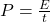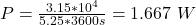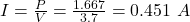## The battery for a certain cell phone is rated at 3.70 V. According to the manufacturer it can produce math]3.15 \times 10^{4} J[/math] of el

Question

The battery for a certain cell phone is rated at 3.70 V. According to the manufacturer it can produce math]3.15 \times 10^{4} J[/math] of electrical energy, enough for 5.25 h of operation, before needing to be recharged. Find the average current that this cell phone draws when turned on.

in progress 0
5 months 2021-08-22T14:28:50+00:00 1 Answers 5 views 0

## Answers ( )

The average current that this cell phone draws when turned on is 0.451 A.

Explanation:

Given;

voltage of the phone, V = 3.7 V

electrical energy of the phone battery, E = 3.15 x 10⁴ J

duration of battery energy, t = 5.25 h

The power the cell phone draws when turned on, is the rate of energy consumption, and this is calculated as follows;where;

P is power in watts

E is energy in Joules

t is time in secondsThe average current that this cell phone draws when turned on:

P = IVTherefore, the average current that this cell phone draws when turned on is 0.451 A.